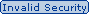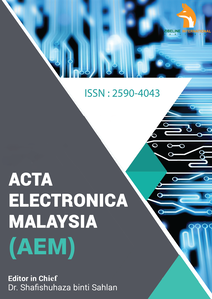# Acta Electronica Malaysia (AEM)

### AN ULTRA-LOW-POWER AND ULTRA-LOW –VOLTAGE 5 GHz LOW NOISE AMPLIFIER DESIGN WITH PRECISE CALCULATION

August 6, 2019 Posted by din In Acta Electronica Malaysia (AEM)

## ABSTRACT

### AN ULTRA-LOW-POWER AND ULTRA-LOW –VOLTAGE 5 GHz LOW NOISE AMPLIFIER DESIGN WITH PRECISE CALCULATION

Acta Electronica Malaysia (AEM)In this paper a low-noise amplifier (LNA) is designed at 5GHz with the intention of ultra-low-power consumption. First, a spiral inductor is discussed and its equivalent circuit is described. Second, the input impedance, output impedance, and gain of a common-source LNA is calculated precisely. In addition, forward body biasing technique is used to bias all transistors to bring down the power consumption of the LNA. Plus, the comparison between precise calculation performed in this paper and the approximation proposed in other papers is demonstrated by HSPICE and MATLAB. The main simulation of the proposed LNA is carried out by Advanced Design System (ADS) and TSMC 0.18 um CMOS process is used for all elements in the LNA. The circuit is evaluated in different voltage supplies from 0.1 volt to 0.5 volt. The LNA is simulated with both lumped-elements and real elements. With lumped-elements the results are 0.96dB, -19dB, -16dB, 17.9dB, and 140μW for noise figure (NF), input impedance matching (S11), output impedance matching (S22), power gain (S21), and power consumption respectively. Plus, with real elements the results are 1.4dB, -20dB, -19dB, 15.4dB, and 139μW for noise figure (NF), input impedance matching (S11), output impedance matching (S22), power gain (S21), and power consumption respectively.